### Learning Outcomes

• Graph stretches and compressions of logarithmic functions.
• Graph reflections of logarithmic functions.

## Graphing Stretches and Compressions of $y=\text{log}_{b}\left(x\right)$

When the parent function $f\left(x\right)={\mathrm{log}}_{b}\left(x\right)$ is multiplied by a constant a > 0, the result is a vertical stretch or compression of the original graph. To visualize stretches and compressions, we set a > 1 and observe the general graph of the parent function $f\left(x\right)={\mathrm{log}}_{b}\left(x\right)$ alongside the vertical stretch, $g\left(x\right)=a{\mathrm{log}}_{b}\left(x\right)$, and the vertical compression, $h\left(x\right)=\frac{1}{a}{\mathrm{log}}_{b}\left(x\right)$.

A General Note: Vertical Stretches and Compressions of the Parent Function $y=\text{log}_{b}\left(x\right)$

For any constant a > 1, the function $f\left(x\right)=a{\mathrm{log}}_{b}\left(x\right)$

• stretches the parent function $y={\mathrm{log}}_{b}\left(x\right)$ vertically by a factor of a if a > 1.
• compresses the parent function $y={\mathrm{log}}_{b}\left(x\right)$ vertically by a factor of a if 0 < a < 1.
• has the vertical asymptote x = 0.
• has the x-intercept $\left(1,0\right)$.
• has domain $\left(0,\infty \right)$.
• has range $\left(-\infty ,\infty \right)$.

The graphs below summarize the key features of the resulting graphs of vertical stretches and compressions of logarithmic functions.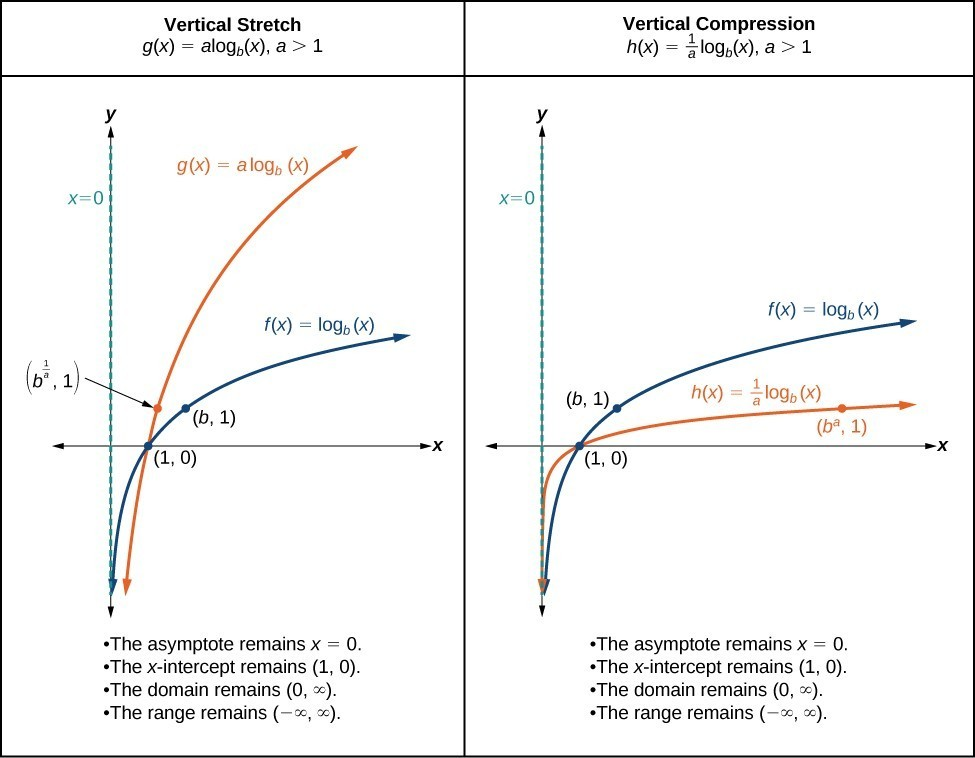How To: Given a logarithmic function Of the form $f\left(x\right)=a{\mathrm{log}}_{b}\left(x\right)$, $a>0$, graph the Stretch or Compression

1. Identify the vertical stretch or compression:
• If $|a|>1$, the graph of $f\left(x\right)={\mathrm{log}}_{b}\left(x\right)$ is stretched by a factor of a units.
• If $|a|<1$, the graph of $f\left(x\right)={\mathrm{log}}_{b}\left(x\right)$ is compressed by a factor of a units.
2. Draw the vertical asymptote x = 0.
3. Identify three key points from the parent function. Find new coordinates for the shifted functions by multiplying the y coordinates in each point by a.
4. Label the three points.
5. The domain is $\left(0,\infty \right)$, the range is $\left(-\infty ,\infty \right)$, and the vertical asymptote is x = 0.

Example: Graphing a Stretch or Compression of the Parent Function $y=\text{log}_{b}\left(x\right)$

Sketch the graph of $f\left(x\right)=2{\mathrm{log}}_{4}\left(x\right)$ alongside its parent function. Include the key points and asymptote on the graph. State the domain, range, and asymptote.

Since the function is $f\left(x\right)=2{\mathrm{log}}_{4}\left(x\right)$, we will note that = 2.

This means we will stretch the function $f\left(x\right)={\mathrm{log}}_{4}\left(x\right)$ by a factor of 2.

The vertical asymptote is x = 0.

Consider the three key points from the parent function, $\left(\frac{1}{4},-1\right)$, $\left(1,0\right)$, and $\left(4,1\right)$.

The new coordinates are found by multiplying the y coordinates of each point by 2.

Label the points $\left(\frac{1}{4},-2\right)$, $\left(1,0\right)$, and $\left(4,\text{2}\right)$.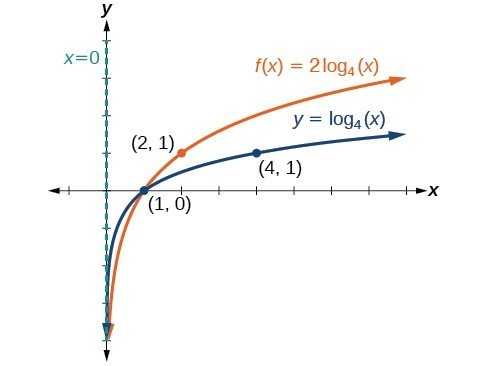The domain is $\left(0,\infty \right)$, the range is $\left(-\infty ,\infty \right)$, and the vertical asymptote is x = 0.

### Try It

Sketch a graph of $f\left(x\right)=\frac{1}{2}{\mathrm{log}}_{4}\left(x\right)$ alongside its parent function. Include the key points and asymptote on the graph. State the domain, range, and asymptote.

The domain is $\left(0,\infty \right)$, the range is $\left(-\infty ,\infty \right)$, and the vertical asymptote is x = 0.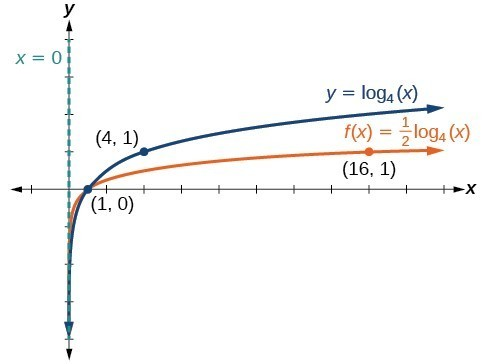### Example: Combining a Shift and a Stretch

Sketch the graph of $f\left(x\right)=5\mathrm{log}\left(x+2\right)$. State the domain, range, and asymptote.

Remember, what happens inside parentheses happens first. First, we move the graph left 2 units and then stretch the function vertically by a factor of 5. The vertical asymptote will be shifted to x = –2. The x-intercept will be $\left(-1,0\right)$. The domain will be $\left(-2,\infty \right)$. Two points will help give the shape of the graph: $\left(-1,0\right)$ and $\left(8,5\right)$. We chose x = 8 as the x-coordinate of one point to graph because when x = 8, x + 2 = 10, the base of the common logarithm.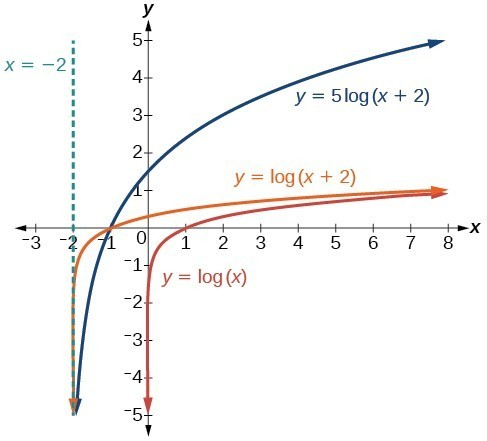The domain is $\left(-2,\infty \right)$, the range is $\left(-\infty ,\infty \right)$, and the vertical asymptote is x = –2.

### Try It

Sketch a graph of the function $f\left(x\right)=3\mathrm{log}\left(x – 2\right)+1$. State the domain, range, and asymptote.

The domain is $\left(2,\infty \right)$, the range is $\left(-\infty ,\infty \right)$, and the vertical asymptote is x = 2.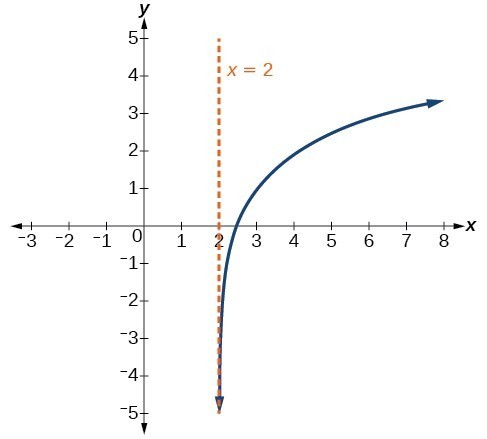## Graphing Reflections of $f\left(x\right)={\mathrm{log}}_{b}\left(x\right)$

When the parent function $f\left(x\right)={\mathrm{log}}_{b}\left(x\right)$ is multiplied by –1, the result is a reflection about the x-axis. When the input is multiplied by –1, the result is a reflection about the y-axis. To visualize reflections, we restrict b > 1 and observe the general graph of the parent function $f\left(x\right)={\mathrm{log}}_{b}\left(x\right)$ alongside the reflection about the x-axis, $g\left(x\right)={\mathrm{-log}}_{b}\left(x\right)$, and the reflection about the y-axis, $h\left(x\right)={\mathrm{log}}_{b}\left(-x\right)$.

### A General Note: Reflections of the Parent Function $y=\text{log}_{b}\left(x\right)$

The function $f\left(x\right)={\mathrm{-log}}_{b}\left(x\right)$

• reflects the parent function $y={\mathrm{log}}_{b}\left(x\right)$ about the x-axis.
• has domain $\left(0,\infty \right)$, range, $\left(-\infty ,\infty \right)$, and vertical asymptote x = 0 which are unchanged from the parent function.

The function $f\left(x\right)={\mathrm{log}}_{b}\left(-x\right)$

• reflects the parent function $y={\mathrm{log}}_{b}\left(x\right)$ about the y-axis.
• has domain $\left(-\infty ,0\right)$.
• has range $\left(-\infty ,\infty \right)$ and vertical asymptote x = 0 which are unchanged from the parent function.

The graphs below summarize the key characteristics of reflecting $f(x) = \log_{b}{x}$ horizontally and vertically.

How To: Given a logarithmic function with the parent function $f\left(x\right)={\mathrm{log}}_{b}\left(x\right)$, graph a Reflection

 $\text{If }f\left(x\right)=-{\mathrm{log}}_{b}\left(x\right)$ $\text{If }f\left(x\right)={\mathrm{log}}_{b}\left(-x\right)$ 1. Draw the vertical asymptote, x = 0. 1. Draw the vertical asymptote, x = 0. 2. Plot the x-intercept, $\left(1,0\right)$. 2. Plot the x-intercept, $\left(1,0\right)$. 3. Reflect the graph of the parent function $f\left(x\right)={\mathrm{log}}_{b}\left(x\right)$ about the x-axis. 3. Reflect the graph of the parent function $f\left(x\right)={\mathrm{log}}_{b}\left(x\right)$ about the y-axis. 4. Draw a smooth curve through the points. 4. Draw a smooth curve through the points. 5. State the domain $\left(0,\infty \right)$, the range $\left(-\infty ,\infty \right)$, and the vertical asymptote x = 0. 5. State the domain, $\left(-\infty ,0\right)$, the range, $\left(-\infty ,\infty \right)$, and the vertical asymptote x = 0.

### Example: Graphing a Reflection of a Logarithmic Function

Sketch a graph of $f\left(x\right)=\mathrm{log}\left(-x\right)$ alongside its parent function. Include the key points and asymptote on the graph. State the domain, range, and asymptote.

Before graphing $f\left(x\right)=\mathrm{log}\left(-x\right)$, identify the behavior and key points for the graph.

• Since b = 10 is greater than one, we know that the parent function is increasing. Since the input value is multiplied by –1, f is a reflection of the parent graph about the y-axis. Thus, $f\left(x\right)=\mathrm{log}\left(-x\right)$ will be decreasing as x moves from negative infinity to zero, and the right tail of the graph will approach the vertical asymptote x = 0.
• The x-intercept is $\left(-1,0\right)$.
• We draw and label the asymptote, plot and label the points, and draw a smooth curve through the points.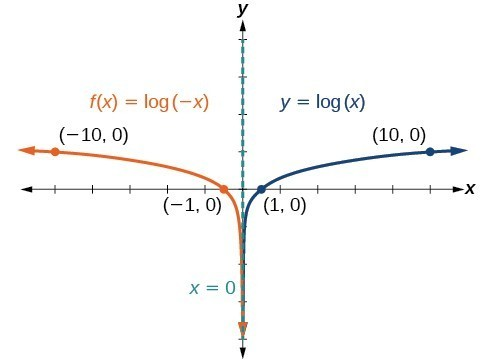The domain is $\left(-\infty ,0\right)$, the range is $\left(-\infty ,\infty \right)$, and the vertical asymptote is x = 0.

### Try It

Graph $f\left(x\right)=-\mathrm{log}\left(-x\right)$. State the domain, range, and asymptote.

The domain is $\left(-\infty ,0\right)$, the range is $\left(-\infty ,\infty \right)$, and the vertical asymptote is x = 0.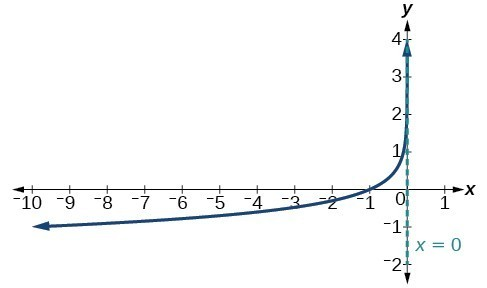How To: Given a logarithmic equation, use a graphing calculator to approximate solutions

1. Press [Y=]. Enter the given logarithmic equation or equations as Y1= and, if needed, Y2=.
2. Press [GRAPH] to observe the graphs of the curves and use [WINDOW] to find an appropriate view of the graphs, including their point(s) of intersection.
3. To find the value of x, we compute the point of intersection. Press [2ND] then [CALC]. Select “intersect” and press [ENTER] three times. The point of intersection gives the value of x for the point(s) of intersection.

### Example: Approximating the Solution of a Logarithmic Equation

Solve $4\mathrm{ln}\left(x\right)+1=-2\mathrm{ln}\left(x – 1\right)$ graphically. Round to the nearest thousandth.

Press [Y=] and enter $4\mathrm{ln}\left(x\right)+1$ next to Y1=. Then enter $-2\mathrm{ln}\left(x – 1\right)$ next to Y2=. For a window, use the values 0 to 5 for x and –10 to 10 for y. Press [GRAPH]. The graphs should intersect somewhere a little to the right of x = 1.

For a better approximation, press [2ND] then [CALC]. Select [5: intersect] and press [ENTER] three times. The x-coordinate of the point of intersection is displayed as 1.3385297. (Your answer may be different if you use a different window or use a different value for Guess?) So, to the nearest thousandth, $x\approx 1.339$.

### Try It

Solve $5\mathrm{log}\left(x+2\right)=4-\mathrm{log}\left(x\right)$ graphically. Round to the nearest thousandth.

$x\approx 3.049$

## Summarizing Transformations of Logarithmic Functions

Now that we have worked with each type of transformation for the logarithmic function, we can summarize each in the table below to arrive at the general equation for transforming exponential functions.

 Transformations of the Parent Function $y={\mathrm{log}}_{b}\left(x\right)$ Translation Form Shift $y={\mathrm{log}}_{b}\left(x+c\right)+d$ Stretch and Compression $y=a{\mathrm{log}}_{b}\left(x\right)$ Reflection about the x-axis $y=-{\mathrm{log}}_{b}\left(x\right)$ Reflection about the y-axis $y={\mathrm{log}}_{b}\left(-x\right)$ General equation for all transformations $y=a{\mathrm{log}}_{b}\left(x+c\right)+d$

### A General Note: Transformations of Logarithmic Functions

All transformations of the parent logarithmic function $y={\mathrm{log}}_{b}\left(x\right)$ have the form

$f\left(x\right)=a{\mathrm{log}}_{b}\left(x+c\right)+d$

where the parent function, $y={\mathrm{log}}_{b}\left(x\right),b>1$, is

• shifted vertically up d units.
• shifted horizontally to the left c units.
• stretched vertically by a factor of |a| if |a| > 0.
• compressed vertically by a factor of |a| if 0 < |a| < 1.
• reflected about the x-axis when a < 0.

For $f\left(x\right)=\mathrm{log}\left(-x\right)$, the graph of the parent function is reflected about the y-axis.

### Example: Finding the Vertical Asymptote of A LogarithmIC Function

What is the vertical asymptote of $f\left(x\right)=-2{\mathrm{log}}_{3}\left(x+4\right)+5$?

The vertical asymptote is at x = –4.

#### Analysis of the Solution

The coefficient, the base, and the upward translation do not affect the asymptote. The shift of the curve 4 units to the left shifts the vertical asymptote to x = –4.

### Try It

What is the vertical asymptote of $f\left(x\right)=3+\mathrm{ln}\left(x – 1\right)$?

### Example: Finding the Equation from a Graph

Find a possible equation for the common logarithmic function graphed below.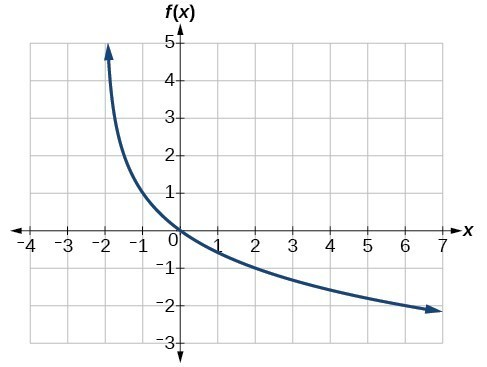This graph has a vertical asymptote at x = –2 and has been vertically reflected. We do not know yet the vertical shift or the vertical stretch. We know so far that the equation will have the form:

$f\left(x\right)=-a\mathrm{log}\left(x+2\right)+k$

It appears the graph passes through the points $\left(-1,1\right)$ and $\left(2,-1\right)$. Substituting $\left(-1,1\right)$,

$\begin{array}{llll}1=-a\mathrm{log}\left(-1+2\right)+k\hfill & \text{Substitute }\left(-1,1\right).\hfill \\ 1=-a\mathrm{log}\left(1\right)+k\hfill & \text{Arithmetic}.\hfill \\ 1=k\hfill & \text{log(1)}=0.\hfill \end{array}$

Next, substituting $\left(2,-1\right)$,

$\begin{array}{llllll}-1=-a\mathrm{log}\left(2+2\right)+1\hfill & \hfill & \text{Plug in }\left(2,-1\right).\hfill \\ -2=-a\mathrm{log}\left(4\right)\hfill & \hfill & \text{Arithmetic}.\hfill \\ \text{ }a=\frac{2}{\mathrm{log}\left(4\right)}\hfill & \hfill & \text{Solve for }a.\hfill \end{array}$

This gives us the equation $f\left(x\right)=-\frac{2}{\mathrm{log}\left(4\right)}\mathrm{log}\left(x+2\right)+1$.

#### Analysis of the Solution

We can verify this answer by comparing the function values in the table below with the points on the graph in this example.

 x −1 0 1 2 3 f(x) 1 0 −0.58496 −1 −1.3219 x 4 5 6 7 8 f(x) −1.5850 −1.8074 −2 −2.1699 −2.3219

### Try It

Give the equation of the natural logarithm graphed below.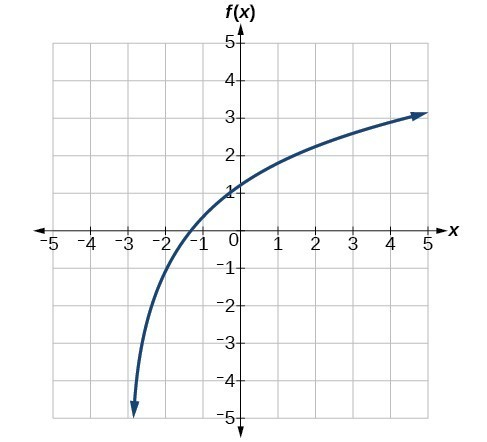### Q & A

Is it possible to tell the domain and range and describe the end behavior of a function just by looking at the graph?

Yes if we know the function is a general logarithmic function. For example, look at the graph in the previous example. The graph approaches x = –3 (or thereabouts) more and more closely, so x = –3 is, or is very close to, the vertical asymptote. It approaches from the right, so the domain is all points to the right, $\left\{x|x>-3\right\}$. The range, as with all general logarithmic functions, is all real numbers. And we can see the end behavior because the graph goes down as it goes left and up as it goes right. The end behavior is as $x\to -{3}^{+},f\left(x\right)\to -\infty$ and as $x\to \infty ,f\left(x\right)\to \infty$.

You are watching: Stretching, Compressing, or Reflecting a Logarithmic Function. Info created by GBee English Center selection and synthesis along with other related topics.